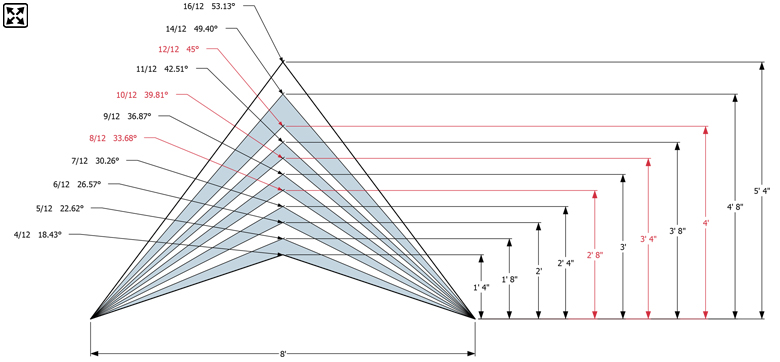# Roof Pitch Chart

The roof's pitch is its vertical rise divided by its horizontal span (or "run"), what is called "slope" in geometry and stair construction, or the tangent function in trigonometry. In imperial measurement systems it is typically expressed with the rise first and run second, with the run denominated by the number 12, giving a ratio of how many inches of incline there is to each 12 inches (one foot) of run. For example, 3:12, 4:12, 5:12, and so on. Architects and designers use a degree angle for computer model input.Roof Pitch Chart with Degree Angle